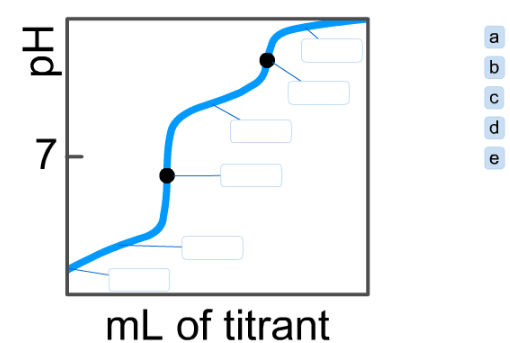# Problem: On the polyprotic weak acid/strong base titration curve below, label the following points. a) The point where the pH corresponds to a solution of H2A in water. b) The point where the pH corresponds to a solution of HA- in water. c) The point where the pH corresponds to a solution of A2- in water. d) The point where pH = pKa1. e) The point where pH = pKa2.

###### FREE Expert Solution

Titration of a Diprotic Acid H2A: H2A ⇌ HA ⇌ A2–

Recall: At halfway of a equivalence point, pH = pKa

90% (204 ratings)###### Problem Details

On the polyprotic weak acid/strong base titration curve below, label the following points.

a) The point where the pH corresponds to a solution of H2A in water.

b) The point where the pH corresponds to a solution of HA- in water.

c) The point where the pH corresponds to a solution of A2- in water.

d) The point where pH = pKa1

e) The point where pH = pKa2.